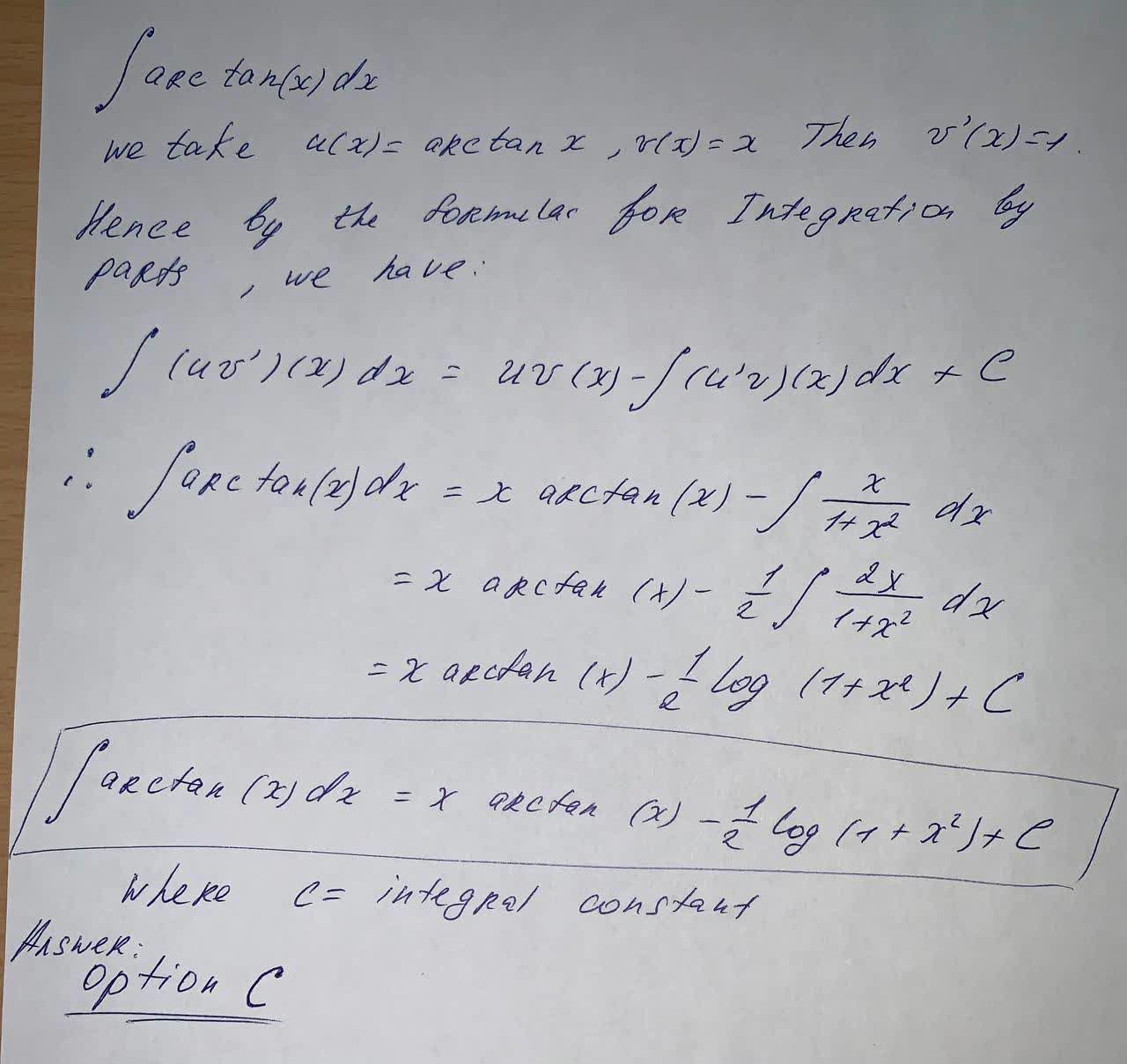#### Didn’t find what you are looking for?

Question# Evaluate the integral \int \arctan(x)dx A)-\arctan(x)+\ln(x^2+1)+C B)\frac{1}{x^2+1}+C C)x \arctan(x)-\frac{1}{2}\ln(x^2+1)+C D) \ln|\arccos(x)|+C E) x\arctan(x)+C

Integrals
ANSWEREDEvaluate the integral $$\int \arctan(x)dx$$
A)$$-\arctan(x)+\ln(x^2+1)+C$$
B)$$\frac{1}{x^2+1}+C$$
C)$$x \arctan(x)-\frac{1}{2}\ln(x^2+1)+C$$
D) $$\ln|\arccos(x)|+C$$
E) $$x\arctan(x)+C$$